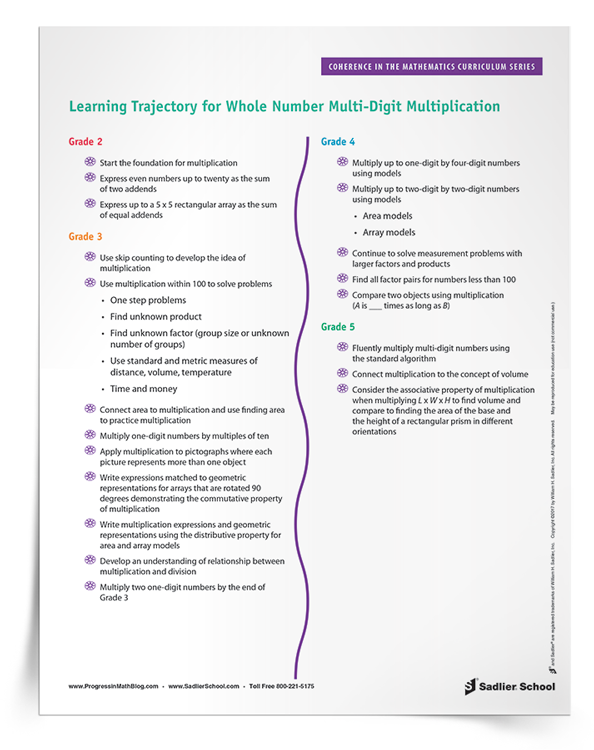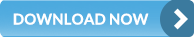Shop Now Login/Register View Quote View Cart
1.800.221.5175
Mathematics
Core Math
Supplemental
Let's Target Math Problem Solving Grades 1–8 View Details | Buy Now
Let's Target Real-World Math Word Problems Grades 1–8 View Details | Buy Now
Critical Thinking for Active Math Minds Grades 3–6 View Details | Buy Now
Vocabulary
Vocabulary Workshop Achieve Interactive Edition Grades 6–12+ View Details | Buy Now
Let's Target Vocabulary and Usage Grades 1–8 View Details | Buy Now
English Language Arts
Grammar & Writing

A K–8 resource to support deep comprehension of math skills and conceptsJanuary 19, 2017 other-professional-development

Whether you are a principal, a math supervisor, a math coach, or a classroom teacher, you may wonder how different elements of the math curriculum are connected between and within the grade levels. That’s why I’m excited about this new monthly series of articles on coherence in the math curriculum. Having a command of how the backbone of the mathematics curriculum is constructed helps support teachers or encourages teachers to support each other while schools help students grow in their mathematics understanding.The monthly downloads for these posts will be a great resource for you in meeting these goals. They are concise, bulleted summaries of what is taught relating to a certain topic across the grades. They also indicate where you can make connections between the different strands of mathematics. The areas of central focus of each grade are supported by additional supporting curricular elements where students can apply their newly-learned knowledge.

If you are using the Common Core State Standards for Mathematics (CCSSM), then your learning trajectory is highly-defined for each grade level. However, even if your school or state has not adopted these standards, you are the resident expert in math curriculum for your school. In this post, we will use example of whole-number multi-digit multiplication in order to explain what I mean about coherence in the curriculum.

One of the things I like best about the CCSSM is that what is taught at each grade level is clear. You can really help teachers maximize their impact by focusing on exactly what needs to be taught. An example of this is the trajectory for learning whole-number multi-digit multiplication:

• Start the foundation for multiplication

• Express even numbers up to twenty as the sum of two addends

• Express up to a 5 x 5 rectangular array as the sum of equal addends

• Use skip counting to develop the idea of multiplication

• Use multiplication within 100 to solve problems

• One-step problems

• Find unknown product

• Find unknown factor (group size or unknown number of groups)

• Use standard and metric measures of distance, volume, temperature

• Time and money

• Connect area to multiplication and use finding area to practice multiplication

• Multiply one-digit numbers by multiples of ten

• Apply multiplication to pictographs where each picture represents more than one object

• Write expressions matched to geometric representations for arrays that are rotated 90 degrees demonstrating the commutative property of multiplication

• Write multiplication expressions and geometric representations using the distributive property for area and array models

• Develop an understanding of relationship between multiplication and division

• Multiply two one-digit numbers by the end of Grade 3

• Multiply up to one-digit by four-digit numbers using models

• Multiply up to two-digit by two-digit numbers using models

• Area models

• Array models

• Continue to solve measurement problems with larger factors and products

• Find all factor pairs for numbers less than 100

• Compare two objects using multiplication (A is ___ times as long as B)

• Fluently multiply multi-digit numbers using the standard algorithm

• Connect multiplication to the concept of volume

• Consider the associative property of multiplication when multiplying L x W x H to find volume and compare to finding the area of the base and the height of a rectangular prism in different orientations

By knowing this trajectory, you can help teachers within each grade level and across grade levels to focus on exactly what to teach. For example, Grade 2 teachers will know to teach the sums of equal addends. Grade 3 teachers can take over and move students toward the memorization of multiplication facts by the end of the year. Grade 4 teachers are responsible for helping students develop array and area models of multiplication, but not necessarily the standard algorithm. This algorithm is to be mastered in Grade 5, though some students may be able to master it earlier. You can help teachers at each grade level understand what their responsibilities are—and what they are not.

In addition, by knowing the trajectory of your curriculum, you can help identify students who are falling behind and design interventions to help them. Thus, second grade students who do not understand the idea of equal addends of rectangular arrays will need help in third grade. Students who do not understand their basic facts by the end of third grade will need help in fourth grade. Early identification and intervention with these students will help everyone, and that’s sure to be part of your job!

Download today’s learning trajectory for multiplication in order to start your collection of coherence maps and stay tuned for subsequent posts so you can better understand the coherence of the mathematics curriculum.